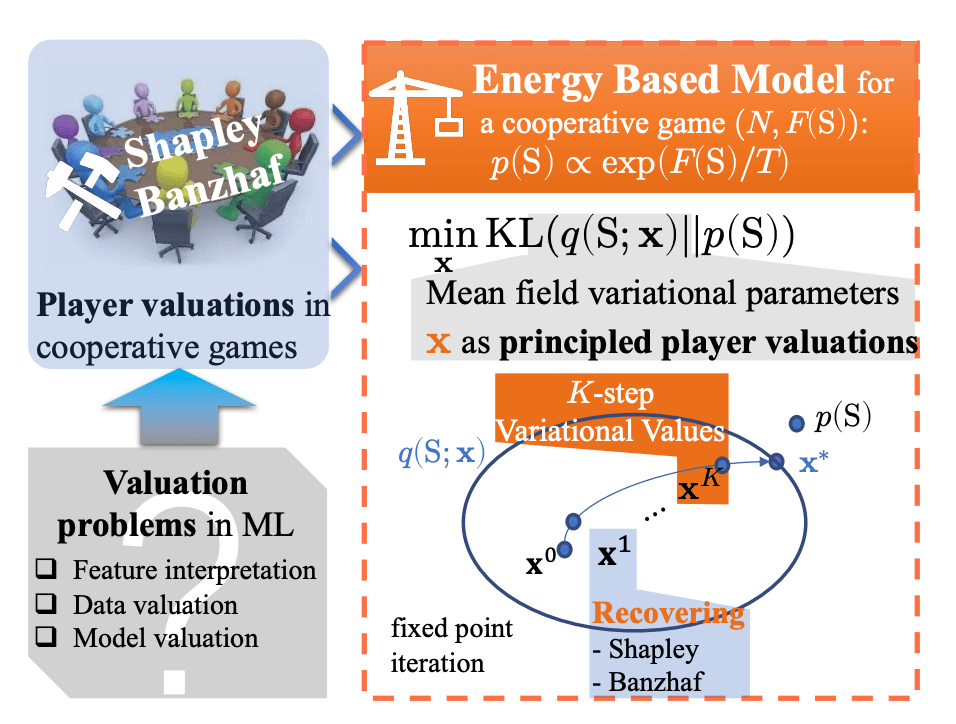Energy-Based Learning for Cooperative Games, with Applications to Valuation Problems in Machine Learning

A theoretical framework connecting data valuation scores, game theory and energy based models is presented. Common criteria like Shapley or Banzhaf can be recovered as special cases.

Valuation problems are gaining more and more popularity in the ML community. Typical examples are feature valuation, i.e. which feature contributes the most to a certain prediction, data valuation, i.e. which data points are more important in model training, or model valuation in ensembles.

Valuation scores are typically formulated using the formalism of cooperative games in game theory. Given a set of players (which could be features or data points in ML), collaborating towards a given task (the training of the model), we want to assign to each a score that represents its overall contribution (how they affect the performance of the model).The paper [Xu21G] shows that such scores can be calculated by maximising the decoupling of players (i.e. effectively breaking their correlations) in a mean-field, energy based model. In the ML setting, the payoff could be the accuracy of a model, and a coalition is any subset of the data or features that we train the model on.

The authors show that their new approach can recover classical criteria of valuation, such as Shapley and Banzhaf values, through a one-step minimisation of the evidence lower bound (ELBO) starting from different initial configurations (different initial weights given to the “players”). More importantly, the authors show that (if initialised uniformly) throughout the minimisation of the ELBO the variational scores satisfy important mathematical properties, such as null value and symmetry, which are key requirements for good valuation scores.

Despite not improving inference time or breaking new state of the art in data valuation, the paper presents a nice theoretical framework that extends the definition of data valuation scores while at the same time drawing the connection to game theory and energy based models.

• Energy-Based Learning for Cooperative Games, with Applications to Valuation Problems in Machine Learning, Yatao Bian, Yu Rong, Tingyang Xu, Jiaxiang Wu, Andreas Krause, Junzhou Huang. (2022)